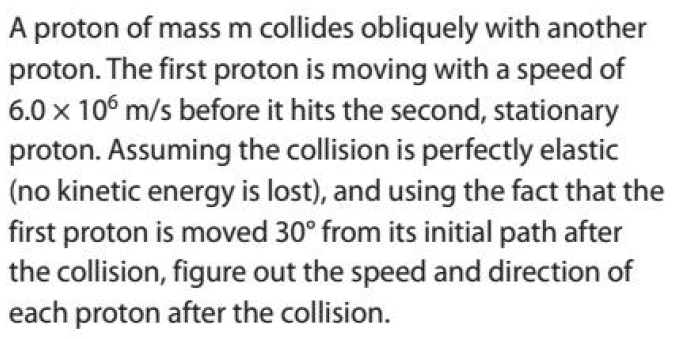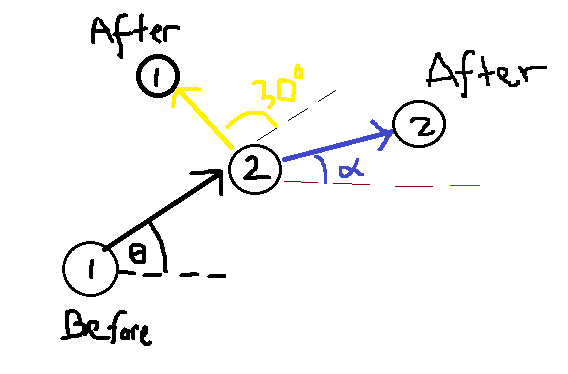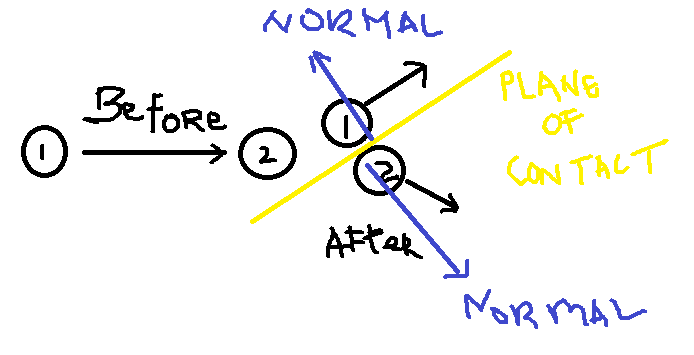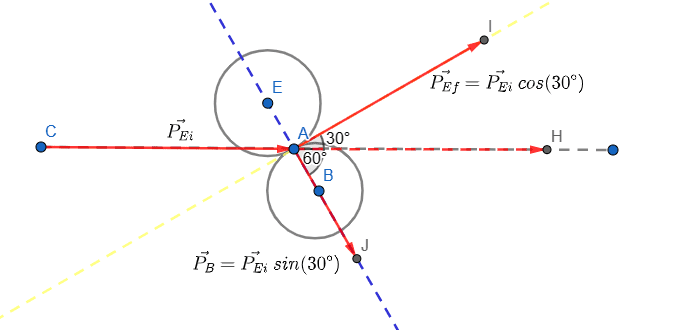# Speed and direction of proton after collision

• songoku

#### songoku

Homework Statement
Relevant Equations
Conservation of momentum

Newton's Experimental LawI don't really understand the question. A proton collides obliquely with another proton means that the first proton moves at certain angle with respect to horizontal?

This is my sketch:Is that correct? If yes, is it solvable since there are so many unknowns?

Thanks

Is that correct? If yes, is it solvable since there are so many unknowns?

Thanks
Yes, it is solvable. You need to calculate the direction of the target proton relative to the initial direction of the incident proton. Your angle ##\theta## is unncessary - you may take ##\theta = 0##.

•songoku
Yes, it is solvable. You need to calculate the direction of the target proton relative to the initial direction of the incident proton. Your angle ##\theta## is unncessary - you may take ##\theta = 0##.
Let:
u1 = initial speed of incident proton = 6 x 106
v1 = final speed of incident proton
v2 = final speed of target proton
α = angle of target proton relative to the initial direction of incident proton

By conservation of momentum in ##x## - direction:
6 x 106 = v1 cos 30o + v2 cos α ... (1)

By conservation of momentum in ##y## - direction:
0 = v1 sin 30o - v2 sin α
v1 = 2 v2 sin α ... (2)

By Newton's experimental Law in ##x## - direction:
6 x 106 = v2 cos α - v1 cos 30o... (3)

From equation (1) and (3), I get v1 = 0 which does not make sense. Do I apply the Newton's Experimental Law correctly?

Thanks

Let:
u1 = initial speed of incident proton = 6 x 106
v1 = final speed of incident proton
v2 = final speed of target proton
α = angle of target proton relative to the initial direction of incident proton

By conservation of momentum in ##x## - direction:
6 x 106 = v1 cos 30o + v2 cos α ... (1)

By conservation of momentum in ##y## - direction:
0 = v1 sin 30o - v2 sin α
v1 = 2 v2 sin α ... (2)

By Newton's experimental Law in ##x## - direction:
6 x 106 = v2 cos α - v1 cos 30o... (3)

From equation (1) and (3), I get v1 = 0 which does not make sense. Do I apply the Newton's Experimental Law correctly?

Thanks
You'd be better using##u_1##.

What you've done for the experimental law doesn't look right at all. You're trying to equate the separation speed of the protons to ##u_1##. That will involve a bit more vector algebra than you've done.

•songoku
Isn't it simpler to use conservation of energy directly?

•songoku
What you've done for the experimental law doesn't look right at all. You're trying to equate the separation speed of the protons to ##u_1##. That will involve a bit more vector algebra than you've done.
What I know about Newton's Experimental Law is:

Coefficient of restitution = ratio of speed of separation to speed of approach

Coefficient of restitution is 1 since it is elastic collision. Is the speed of approach not equal to u1? I thought speed of approach is u1 since target proton is initially at rest.

Isn't it simpler to use conservation of energy directly?
I will try using conservation of energy but right now I want to learn using conservation of momentum to solve this question.

Thanks

I will try using conservation of energy but right now I want to learn using conservation of momentum to solve this question.

Thanks
You need both.

•SolarisOne, Steve4Physics and songoku
You need both.
I just tried using conservation of kinetic energy and I am able to do this question.

If I want to use Newton's Experimental Law, what is the correct way to do it?
The speed of separation of the protons is v2 cos α - v1 cos 30o but the speed of approach is not u1?

Thanks

I just tried using conservation of kinetic energy and I am able to do this question.

If I want to use Newton's Experimental Law, what is the correct way to do it?
The speed of separation of the protons is v2 cos α - v1 cos 30o but the speed of approach is not u1?

Thanks
To get the separation speed after the collision, you have to take the modulus of the difference of the velocity vectors.

•songoku
I just tried using conservation of kinetic energy and I am able to do this question.
Unlikely! You need both conservation of (kinetic) energy and conservation of momentum to solve the problem, as already noted by @PeroK. Conservation of kinetic energy alone does not account for directions. If you show your working we should be able to check it.

If I want to use Newton's Experimental Law, what is the correct way to do it?
The speed of separation of the protons is v2 cos α - v1 cos 30o but the speed of approach is not u1?
Newton's experimental law deals with relative speeds of approach and separation, not velocities. So, by itself, it does not allow you to determine directions. You still need conservation of momentum. What can you say about the initial and final relative speeds in this problem?

Do you know how to change to/from the centre-of-mass frame of reference (which often simplifies collision problems)?

Also note, in Post #1:
- the angle marked as 30º looks more like (about) 100º!
- there is no reference to ‘the horizontal’ in the original question; for convenience, we typically choose to have the incident photon traveling along the x-axis (in the +x direction) - the same as using θ=0 as already suggested by @PeroK;
- the angle marked α is not the one you should be trying to find!
If you need further help you might want to redo and repost the diagram as it is misleading as it stands.

•songoku
A bit of experience playing pool is useful. In a collision between the cue ball and a target ball there is a simple relationship between the angles at which the cue ball and the target ball depart from the collision.

•hutchphd, Steve4Physics and songoku
To get the separation speed after the collision, you have to take the modulus of the difference of the velocity vectors.
Now I see why you said it is simpler to use conservation of energy directly. I follow your hint to find separation speed and the algebra becomes quite messy. Maybe if I continue working on it I will get the same equation as conservation of KE

Unlikely! You need both conservation of (kinetic) energy and conservation of momentum to solve the problem, as already noted by @PeroK. Conservation of kinetic energy alone does not account for directions. If you show your working we should be able to check it.
Sorry, I mean I used both conservation of momentum and KE. In my previous working I used conservation of momentum and Newton's experimental law (wrongly).

Newton's experimental law deals with relative speeds of approach and separation, not velocities. So, by itself, it does not allow you to determine directions.
I have notes stating that for elastic head-on collisions, relative velocity of approach = - relative velocity of separation or relative speed of approach = relative speed of separation.

That's why I thought I could apply Newton's Experimental Law to relative velocity (or relative speed) for ##x## and ##y## direction separately, which turns out to be wrong.

Is this because it is only for head-on collisions? Is head-on collision meant that no angle involved in the collision?

I think it is better for me to stick with relative speed rather than relative velocity

Do you know how to change to/from the centre-of-mass frame of reference (which often simplifies collision problems)?
I don't. I want to learn about it but I can't find book explaining about it

Also note, in Post #1:
- the angle marked α is not the one you should be trying to find!
Yes, I realized it from post#2, that's why I changed the position of the angle in post#3 but sorry I didn't change the sketch.

Thanks

•Steve4Physics
Now I see why you said it is simpler to use conservation of energy directly. I follow your hint to find separation speed and the algebra becomes quite messy. Maybe if I continue working on it I will get the same equation as conservation of KE
It shouldn't be too messy. You have ##\vec v_1 = (v_1\cos 30, v_1 \sin 30)## and ##\vec v_2 = (v_2 \cos \alpha, -v_2 \sin \alpha)##. Just subtract those, and using conservation of energy gives you the angle ##\alpha##. Interesting!

•songoku
It shouldn't be too messy. You have ##\vec v_1 = (v_1\cos 30, v_1 \sin 30)## and ##\vec v_2 = (v_2 \cos \alpha, -v_2 \sin \alpha)##. Just subtract those, and using conservation of energy gives you the angle ##\alpha##. Interesting!
Or use the Pythagorean theorem and geometry.

Call the impacting velocity ##v_0## and the departure velocities ##v_1## and ##v_2##. By conservation of momentum, ##\vec{v_0} = \vec{v_1} + \vec{v_2}##. This means that the three vectors form a triangle.

By conservation of energy, ##\frac{1}{2}mv_0^2=\frac{1}{2}mv_1^2+\frac{1}{2}mv_2^2##. Divide out the ##\frac{1}{2}m##. Now you have ##v_0^2=v_1^2+v_2^2##.

But that's the Pythagorean theorem for right triangles. If the triangle is a right triangle, the sides have that relationship. And conversely. If the sides have that relationship then the triangle is right.

Which brings us back to the pool table and those balls departing at a particular angle from one another.

•songoku, Steve4Physics and PeroK
I have notes stating that for elastic head-on collisions, relative velocity of approach = - relative velocity of separation or relative speed of approach = relative speed of separation.

That's why I thought I could apply Newton's Experimental Law to relative velocity (or relative speed) for ##x## and ##y## direction separately, which turns out to be wrong.

Is this because it is only for head-on collisions? Is head-on collision meant that no angle involved in the collision?
Yes. For a head-on elastic collision between 2 particles:
<relative velocity of approach> = - <relative velocity of separation>

That's the 1D (1-dimensional) case - where all velocities lie on the same straight line. Of course, as a consequence):
(relative speed of approach) = (relative speed of separation).

But for an oblique elastic collision (the 2D case - velocities in the same plane) <relative velocity of approach> is not equal to -<relative velocity of separation>. Can you imagine sitting on one of the protons and observing the direction of the other proton before and after the collision?

But, interestingly, in the 2D case we can still say:
(relative speed of approach) = (relative speed of separation)
Though this is not necessarily the best way to tackle this particular problem.

... I want to learn about it [using the centre-of-mass frame of reference] but I can't find book explaining about it
There are a number of Youtube videos relating to using the centre-of-mass frame of reference for solving collision problems. I can't recommend a particular video (and I don't know your level of background knowledge) but take a look for yourself if interested.

•songoku
Newton's experimental law deals with relative speeds of approach and separation, not velocities.
Well, no, it does deal with velocities, but it is the relative velocity components normal to the plane of contact. I've always used it in conjunction with momentum rather than using energy + momentum because it avoids the quadratics.

•songoku
Well, no, it does deal with velocities, but it is the relative velocity components normal to the plane of contact. I've always used it in conjunction with momentum rather than using energy + momentum because it avoids the quadratics.
Surely Newton’s Experimental Law (NEL) specifically refers to the magnitudes of relative velocities, which are speeds.

As I understand it (though I could be wrong) NEL essentially states that for a pair of colliding bodies:
speed of separation ∝ speed of approach
(the constant of proportionality being the coefficient of restitution).

But I agree that the relevant speeds must be the magnitudes of the components of relative velocities in the appropriate direction - the direction being that of the common normal at the point impact.

•songoku
Surely Newton’s Experimental Law (NEL) specifically refers to the magnitudes of relative velocities, which are speeds.

As I understand it (though I could be wrong) NEL essentially states that for a pair of colliding bodies:
speed of separation ∝ speed of approach
(the constant of proportionality being the coefficient of restitution).

But I agree that the relevant speeds must be the magnitudes of the components of relative velocities in the appropriate direction - the direction being that of the common normal at the point impact.
If it only depended on the relative speeds there would be an ambiguity over the sign of the post collision relative velocity.

•songoku
If it only depended on the relative speeds there would be an ambiguity over the sign of the post collision relative velocity.
You’ve partly convinced me. But note that when expressing NEL as:
speed of separation ∝ speed of approach
the terms 'separation' and 'approach' embody the sign information (the directions). So NEL can be correctly expressed this way without the explicit use of vectors.

I can't find a formal definition of NEL but would be interested to see one.

•songoku
expressing NEL as:
speed of separation ∝ speed of approach
That still needs to be prefixed by specifying the components normal to the contact plane. And the sign only becomes clear by assuming the bodies can't pass through each other. Using velocities it's embodied in the equation.

•songoku
That still needs to be prefixed by specifying the components normal to the contact plane. And the sign only becomes clear by assuming the bodies can't pass through each other. Using velocities it's embodied in the equation.
Agreed.

It shouldn't be too messy. You have ##\vec v_1 = (v_1\cos 30, v_1 \sin 30)## and ##\vec v_2 = (v_2 \cos \alpha, -v_2 \sin \alpha)##. Just subtract those, and using conservation of energy gives you the angle ##\alpha##. Interesting!
I subtract then find the modulus:

$$|\vec v_1 - \vec v_2|=\sqrt{(v_1 \cos 30-v_2 \cos \alpha)^2+(v_1 \sin 30+v_2 \sin \alpha)^2}$$
$$=\sqrt{(v_1 \cos 30)^2-2v_1 v_2 \cos 30 \cos \alpha+(v_2 \cos \alpha)^2+(v_1 \sin 30)^2+2v_1 v_2 \sin 30 \sin \alpha +(v_2 \sin \alpha)^2}$$
$$=\sqrt{(v_1)^2+(v_2)^2-\sqrt{3} v_1 v_2 \cos \alpha+v_1 v_2 \sin \alpha}$$

Equating this to relative speed of approach (which is ##u_1##):
$$(u_1)^2=(v_1)^2+(v_2)^2-\sqrt{3} v_1 v_2 \cos \alpha+v_1 v_2 \sin \alpha$$

Comparing to the equation I get from conservation of KE (##u_1^2=v_1^2+v_2^2##) , there are two more terms. After finding the angle ##\alpha## using conservation of momentum and KE, actually those two terms will cancel out but I am not sure how to cancel those without finding the angle first.

Or use the Pythagorean theorem and geometry.

Call the impacting velocity ##v_0## and the departure velocities ##v_1## and ##v_2##. By conservation of momentum, ##\vec{v_0} = \vec{v_1} + \vec{v_2}##. This means that the three vectors form a triangle.

By conservation of energy, ##\frac{1}{2}mv_0^2=\frac{1}{2}mv_1^2+\frac{1}{2}mv_2^2##. Divide out the ##\frac{1}{2}m##. Now you have ##v_0^2=v_1^2+v_2^2##.

But that's the Pythagorean theorem for right triangles. If the triangle is a right triangle, the sides have that relationship. And conversely. If the sides have that relationship then the triangle is right.

Which brings us back to the pool table and those balls departing at a particular angle from one another.
First time I see this type of approach and this is brilliant

A bit of experience playing pool is useful. In a collision between the cue ball and a target ball there is a simple relationship between the angles at which the cue ball and the target ball depart from the collision.
You mean the angle between those two balls will always be 90 degrees?

There are a number of Youtube videos relating to using the centre-of-mass frame of reference for solving collision problems. I can't recommend a particular video (and I don't know your level of background knowledge) but take a look for yourself if interested.
I will take a look and try to redo this question by using that method

Well, no, it does deal with velocities, but it is the relative velocity components normal to the plane of contact.
By normal to the plane of contact, do you mean this?Thanks

By normal to the plane of contact, do you mean this?
View attachment 290668
Yes.
The impulse is normal to the plane of contact. (For spheres, that means it is along the line joining the centres, but that is not so in general.)
So neither body will undergo a change in velocity parallel to the plane of contact, and you can apply momentum conservation and NEL normal to it. That also applies for collisions that are not perfectly elastic.

•songoku
You mean the angle between those two balls will always be 90 degrees?
Yes.

•songoku
Thank you very much PeroK, Steve4Physics, jbriggs444, haruspex

Thank you very much PeroK, Steve4Physics, jbriggs444, haruspex
Ok.
As you might know, for a perfectly elastic full on collision between two identical balls, one starting at rest, the incoming ball comes to rest. Applying what I wrote in post #23, it follows that in an oblique collision the incoming ball loses all its velocity normal to the plane of contact. Hence, the balls will move off at right angles to each other.

•songoku•songoku
Thank you very much neilparker62 for such a nice sketch

Thank you very much neilparker62 for such a nice sketch
Pleasure - hope it helps understand the problem! Geometer's sketchpad is a great online tool for this type of sketch.

•songoku